## Alternating guided image filteringRT @PeerJCompSci: Alternating guided image filtering https://t.co/Qbp3PjsLg0 https://t.co/GEf2WI3sEqAlternating guided image filtering https://t.co/Qbp3PjsLg0 https://t.co/GEf2WI3sEq

## Introduction

Natural scenes contain meaningful visual elements at different spatial scales. Small elements typically represent texture, small objects, and noise, while large scale structures generally represent object or region boundaries, spatial color transitions, or homogeneous regions. Spatial filtering is a common operation in image processing and computer vision that is typically used to reduce noise or eliminate small spurious details (e.g., texture) and enhance image contrast. In spatial filtering, the value of the filtered image at a given location is a function of the original pixel values in a small neighborhood of the same location. In linear filtering, the value of an output pixel is a linear combination (e.g., a weighted average) of the values of the pixels in the input pixel’s neighborhood. When the image intensity varies smoothly over space, nearby pixels are likely to have similar values (i.e., they will be correlated). In contrast, the noise that corrupts these pixel values will be less correlated than the signal values, so that averaging reduces noise while preserving the mean signal value. However, the assumption of smooth variation evidently does not hold near edges. Thus, although linear filtering can effectively reduce image noise and eliminate unwanted details smaller than the filter kernel size, it degrades the articulation of (blurs) the remaining edges, lines and other details that are important for the interpretation of the image. Therefore, edge preserving filters have been developed that reduce small (relative to the filter kernel size) scale image variations (noise or texture) while preserving larger scale discontinuities (edges).

Some well-known non-linear edge-preserving smoothing filters are for instance anisotropic diffusion (Perona & Malik, 1990), robust smoothing (Black et al., 1998) and the bilateral filter (Tomasi & Manduchi, 1998). However, anisotropic diffusion tends to oversharpen edges (i.e., it produces halos) and is computationally expensive, which makes it less suitable for application, for instance, in multiresolution schemes (Farbman et al., 2008). The non-linear bilateral filter (BLF) assigns each pixel a weighted mean of its neighbors, with the weights decreasing both with spatial distance and with difference in value (Tomasi & Manduchi, 1998). While the BLF is quite effective at smoothing small intensity changes while preserving strong edges and has efficient implementations, it also tends to blur across edges at larger spatial scales, thereby limiting its value for application in multiscale image decomposition schemes (Farbman et al., 2008). In addition, the BLF has the undesirable property that it can reverse the intensity gradient near sharp edges (the weighted average becomes unstable when a pixel has only few similar pixels in its neighborhood: He, Sun & Tang, 2013). In the joint (or cross) bilateral filter (JBLF) a second or guidance image serves to steer the edge stopping range filter thus preventing over- or under- blur near edges (Petschnigg et al., 2004). The recently-introduced Guided Filter (GF: He, Sun & Tang, 2013) is a computationally efficient, edge-preserving translation-variant operator based on a local linear model which avoids the drawbacks of bilateral filtering and other previous approaches. When the input image also serves as the guidance image, the GF behaves like the edge preserving BLF. However, similar to the BLF, the GF also tends to produce halos near significant edges.

Two recently presented iterative guided filtering frameworks have the ability to perform edge preserving filtering without the introduction of halos. Zhang et al. (2014) showed that the application of the JBLF in an iterative Rolling Guided Filter (RGF) framework results in size selective filtering of small scale details combined with the recovery of larger scale edges. However, the RGF also has a drawback: it tends to smooth the curvature of large scale edges. Kniefacz & Kropatsch (2015) recently introduced a similar framework called the Smooth and iteratively Restore (SiR) filter. SiR restores large scale edges while preserving their curvature. However, SiR also has two drawbacks: it reduces the local image intensity and restores small scale details in the neighborhood of large scale edges.

In this paper we propose an Alternating Guided Filter (AGF) scheme that integrates the RGF and SiR in a single framework, resulting in a new image smoothing operator that preserves significant edges while effectively eliminating small scale details. AGF combines the edge preserving properties of both the RGF (i.e., the elimination of small details in combination with local intensity preservation) and the SiR (i.e., the articulated restoration of large scale edges) while it does not suffer from their respective drawbacks (the curvature smoothing of large scale edges by RGF and local intensity reduction in combination with the restoration of small scale details near large scale edges by SiR).

The rest of this paper is organized as follows. ‘Related Work’ briefly discusses some related work on edge preserving filtering and introduces the RGF and SiR filter techniques on which the proposed AGF scheme is based. ‘Alternating Guided Filter’ presents the proposed Alternating Guided Filter scheme. ‘Experimental Results’ presents the results of the application of the AGF filter to natural and synthetic images, compares the performance of this new framework with the RGF and SiR filter schemes, and provides some runtime estimates. Finally, in ‘Conclusions’ the conclusions of this study are presented.

## Related Work

In this section we briefly review the edge preserving bilateral and joint bilateral filters and show how they are related to the guided filter. We also discuss two recently introduced frameworks that iteratively apply joint bilateral filters to achieve selective guided filtering of small scale details in combination with large scale edge restoration.

### Bilateral filter

The bilateral filter is a non-linear filter that computes the output at each pixel as a Gaussian weighted average of their spatial and intensity distances. It prevents blurring across edges by assigning larger weights to pixels that are spatially close and have similar intensity values (Tomasi & Manduchi, 1998). It uses a combination of (typically Gaussian) spatial and a range (intensity) filter kernels that perform a blurring in the spatial domain weighted by the local variation in the intensity domain. It combines a classic low-pass filter with an edge-stopping function that attenuates the filter kernel weights at locations where the intensity difference between pixels is large. Bilateral filtering was developed as a fast alternative to the computationally expensive technique of anisotropic diffusion, which uses gradients of the filtering images itself to guide a diffusion process, thus avoiding edge blurring (Perona & Malik, 1990). More formally, at a given image location (pixel) i, the filtered output Oi is given by: ${O}_{i}=\frac{1}{{K}_{i}}\sum _{j\in \mathrm{\Omega }}{I}_{j}f\left(\parallel i-j\parallel \right)g\left(\parallel {I}_{i}-{I}_{j}\parallel \right)$ where f is the spatial filter kernel (e.g., a Gaussian centered at i), g is the range or intensity (edge-stopping) filter kernel (centered at the image value at i), $\mathrm{\Omega }$ is the spatial support of the kernel, Ki is a normalizing factor (the sum of the fg filter weights).

The bilateral filter is controlled by only two parameters: the extent of respectively the spatial kernel and the range kernel. Intensity edges are preserved since the bilateral filter decreases not only with the spatial distance but also with the intensity distance. Though the filter has efficient implementations (Paris & Durand, 2006) and effectively reduces noise while preserving edges in many situations, it has the undesirable property that it can reverse the intensity gradient near sharp edges (the weighted average becomes unstable when a pixel has only few similar pixels in its neighborhood (He, Sun & Tang, 2013)).

In the joint (or cross) bilateral filter (JBLF) the range filter g is applied to a second or guidance image G (Petschnigg et al., 2004): ${O}_{i}=\frac{1}{{K}_{i}}\sum _{j\in \mathrm{\Omega }}{I}_{j}\cdot f\left(\parallel i-j\parallel \right)\cdot g\left(\parallel {G}_{i}-{G}_{j}\parallel \right).$

The JBLF can prevent over- or under- blur near edges by using a related image G to guide the edge stopping behavior of the range filter. That is, the JBLF smooths the image I while preserving edges that are also represented in the image G. The JBLF is particularly favored when the edges in the image that is to be filtered are unreliable (e.g., due to noise or distortions) and when a companion image with well-defined edges is available (e.g., in the case of flash /no-flash image pairs).

### Guided Filter

A Guided Filter (GF: He, Sun & Tang, 2013) is a translation-variant filter based on a local linear model. Guided image filtering involves an input image I, a guidance image G (which may be identical to the input image), and an output image O. The two filtering conditions are (i) that the local filter output is a linear transform of the guidance image G and (ii) as similar as possible to the input image I. The first condition implies that ${O}_{i}={a}_{k}{G}_{i}+{b}_{k}\phantom{\rule{10.00002pt}{0ex}}\forall i\in {\omega }_{k}$ where ωk is a square window of size (2r + 1) × (2r + 1). The local linear model ensures that the output image O has an edge only at locations where the guidance image G has one, because ∇O = aG. The linear coefficients ak and bk are constant in ωk. They can be estimated by minimizing the squared difference between the output image O and the input image I (the second filtering condition) in the window ωk, i.e., by minimizing the cost function E: $E\left({a}_{k},{b}_{k}\right)=\sum _{i\in {\omega }_{k}}\left({\left({a}_{k}{G}_{i}+{b}_{k}-{I}_{i}\right)}^{2}+\epsilon {a}_{k}^{2}\right)$ where ε is a regularization parameter penalizing large ak. The coefficients ak and bk can directly be solved by linear regression (He, Sun & Tang, 2013): ${a}_{k}=\frac{\frac{1}{|\omega |}\sum _{i\in {\omega }_{k}}{G}_{i}{I}_{i}-{\overline{G}}_{k}{\overline{I}}_{k}}{{\sigma }_{k}^{2}+\epsilon }$ ${b}_{k}={\overline{I}}_{k}-{a}_{k}{\overline{G}}_{k}$

where |ω| is the number of pixels in ωk, ${\overline{I}}_{k}$ and ${\overline{G}}_{k}$ represent the means of respectively I and G over ωk, and ${\sigma }_{k}^{2}$ is the variance of I over ωk.

Since pixel i is contained in several different (overlapping) windows ωk, the value of Oi in Eq. (3) depends on the window over which it is calculated. This can be accounted for by averaging over all possible values of Oi: ${O}_{i}=\frac{1}{|\omega |}\sum _{k|i\in {\omega }_{k}}\left({a}_{k}{G}_{k}+{b}_{k}\right).$ Since ∑k|iωkak = ∑kωiak due to the symmetry of the box window Eq. (7) can be written as ${O}_{i}={\overline{a}}_{i}{G}_{i}+{\overline{b}}_{i}$ where ${\overline{a}}_{i}=\frac{1}{|\omega |}{\sum }_{k\in {\omega }_{i}}{a}_{k}$ and ${\overline{b}}_{i}=\frac{1}{|\omega |}{\sum }_{k\in {\omega }_{i}}{b}_{k}$ are the average coefficients of all windows overlapping i. Although the linear coefficients $\left({\overline{a}}_{i},{\overline{b}}_{i}\right)$ vary spatially, their gradients will be smaller than those of G near strong edges (since they are the output of a mean filter). As a result we have $\nabla O\approx \overline{a}\nabla G$, meaning that abrupt intensity changes in the guiding image G are still largely preserved in the output image O.

Equations (5), (6), and (8) define the GF. When the input image also serves as the guidance image, the GF behaves like the edge preserving bilateral filter, with the parameters ε and the window size r having the same effects as respectively the range and the spatial variances of the bilateral filter. Equation (8) can be rewritten as ${O}_{i}=\sum _{j}{W}_{ij}\left(G\right){I}_{j}$ with the weighting kernel Wij depending only on the guidance image G: ${W}_{ij}=\frac{1}{{|\omega |}^{2}}\sum _{k:\left(i,j\right)\in {\omega }_{k}}\left(1+\frac{\left({G}_{i}-{\overline{G}}_{k}\right)\left({G}_{j}-{\overline{G}}_{k}\right)}{{\sigma }_{k}^{2}+\epsilon }\right).$ Since ∑jWij(G) = 1 this kernel is already normalized.

The GF is a computationally efficient, edge-preserving operator which avoids the gradient reversal artefacts (i.e., over sharpening of edges) of the BLF. However, just like the BLF, GF has the limitation that it tends to produce halos (unwanted blurring) near large scale edges (He, Sun & Tang, 2013).

### Rolling Guidance Filter

Zhang et al. (2014) showed that the application of the joint bilateral filter (Eq. (2)) in an iterative framework results in effective size selective filtering of small scale details combined with the recovery of larger scale edges. In their Rolling Guidance Filter (RGF) framework the result Gt+1 of the tth iteration is obtained from the joint bilateral filtering of the input image I using the result Gt of the previous iteration step as the guidance image: ${G}_{i}^{t+1}=\frac{1}{{K}_{i}}\sum _{j\in \mathrm{\Omega }}{I}_{j}\cdot f\left(\parallel i-j\parallel \right)\cdot g\left(\parallel {G}_{i}^{t}-{G}_{j}^{t}\parallel \right).$ In the RGF scheme details smaller than the Gaussian kernel of the bilateral filter are initially removed while the edges of the remaining details are restored by iteratively updating the guidance image. At the start of the iteration process the term $\parallel {G}_{i}^{t}-{G}_{j}^{t}\parallel$ is almost zero, making the range filter g inoperative, so that the joint bilateral filter effectively behaves like a Gaussian filter. Details removed by this filter cannot be recovered later in the process. After each iteration step the influence of the range filter gradually increases due to its increasing weights. Hence, guided by the input image I, the RGF scheme gradually restores the edges that remain after the initial Gaussian filtering. Note that the initial guidance image G1 can simply be a constant (e.g., zero) valued image since it updates to the Gaussian filtered input image in the first iteration step. The pseudocode for the RGF scheme is presented in Table 1.

The RGF scheme is not restricted to the JBF (Zhang et al., 2014). Any average-based joint edge aware averaging filter (e.g., the Guided Filter) can be applied in the RGF framework. In practice the RGF converges rapidly after only a few (3–5) iterations steps. The RGF scheme is simple to implement and can be efficiently computed.

Although the RGF enables effective and efficient size selective filtering, it has as drawback that the remaining large scale edges are smoothly curved.

### Smooth and iteratively Restore filter

Kniefacz & Kropatsch (2015) recently introduced the Smooth and iteratively Restore (SiR) filter. Similar to RGF, SiR initially removes small scale details (e.g., through Gaussian filtering) and uses an edge-aware filter to iteratively restore larger scale edges. Whereas RGF iteratively filters the (fixed) input image while iteratively restoring the initially blurred guidance image, SiR does the opposite: it gradually restores the initially blurred image using the original input image as (fixed) guidance. Thereto, small details are initially removed through blurring with a Gaussian kernel: ${G}_{i}^{0}=\frac{1}{K}\sum _{j\in \mathrm{\Omega }}{I}_{j}\cdot f\left(\parallel i-j\parallel \right).$ Then, the edges of the remaining details are iteratively restored by repeatedly computing an updated image Gt+1 by joint bilateral filtering of the blurred image Gt using the input image I as the guidance image: ${G}_{i}^{t+1}=\frac{1}{{K}_{i}}\sum _{j\in \mathrm{\Omega }}{G}_{j}^{t}\cdot f\left(\parallel i-j\parallel \right)\cdot g\left(\parallel {I}_{i}-{I}_{j}\parallel \right).$ Similar to RGF, SiR converges rapidly after only a few (3–5) iterations steps. Compared to RGF, SiR has the advantage that it produces edges with articulated curvature, since it attempts to restore them as similar as possible to the edges in the original image. In contrast, the curvature of edges produced by RGF is smoothed (less articulated).

SiR also has two drawbacks (Kniefacz & Kropatsch, 2015). First, the overall intensity of the result is less than the overall intensity of the original image, since SiR restores edges starting from the initially blurred input image. RGF does not have this problem because it operates on the original image itself. Second, SiR tends to restore small scale details that are close to large scale edges. This is a result of a spill-over effect (blurring) of the large scale edges which enables the JBF (Eq. (13)) to restore small scale details guided by the original input image. As will be shown in ‘Relative performance of SiR and SiRmed’ this restoration of small details near larger edges by SiR can effectively be suppressed by applying a median filter at the end of each iteration step with a kernel that is smaller than the Gaussian kernel of the bilateral spatial filter (e.g., 3 × 3). At each iteration step the median filer “cleans” the image by removing small details that are recovered by the bilateral filter. In the rest of this paper, we will refer to this modification of the original SiR algorithm as SiRmed (Smooth and iteratively Restore including median filtering). The pseudocode for the SiRmed algorithm is presented in Table 2. The pseudocode for the SiR algorithm is obtained by omitting Step 5 (the median filter).

## Alternating Guided Filter

In this section we propose a new filter scheme that effectively integrates the RGF and SiR frameworks in such a way that it retains the desired effects of both earlier schemes (i.e., the elimination of small details and restoration of large scale edges) while eliminating their respective drawbacks (i.e., the smoothing of the curvature of large scale edges by RGF and the loss of local image intensity by SiR). Each iteration in the AGF framework consists of three consecutive steps. First, joint bilateral filtering is applied to the input image I, using the result Gt of the previous iteration step as the guidance image (Eq. (11)). Second, joint bilateral filtering is applied to the result from the first step, using the original image I as the guidance image (Eq. (13)). Third, a median filter with a small kernel size (e.g., 3 × 3) is applied to the result of the second step. The alternating use of the original image and the filtered result as either input or guidance images guarantees that both the overall image intensity (positive effect of the RGF scheme) and the curvature of the large scale edges (positive effect of the SiR scheme) are preserved, while the integration of the median filter prevents the reintroduction of filtered small scale details near large scale edges (a negative side effect of SiR). Hence, the AGF scheme combines the positive effects of both the RGF (intensity preservation) and SiR (edge curvature preservation) while eliminating their negative side effects (edge curvature smoothing by the RGF scheme and contrast reduction plus the reintroduction of small details near larger edges by the SiR scheme). The pseudo code for the AGF scheme is presented in Table 3.

## Experimental Results

In this section we present the results of some experiments that were performed to study the performance of the proposed AGF framework. We tested AGF by applying it to a wide range of different natural images (for an overview of all results see the Supplemental Information with this paper). Also, we compare the performance of AGF with that of RGF and SiRmed.

The images used in this study have a width of 638 pixels, and a height that varies between 337 and 894 pixels. We selected a set of test images with widely varying features and statistics, such as landscapes and aerial images, portraits and mosaics, embroidery and patchwork. The full set of test images with the results of the different filter schemes are provided as Supplemental Information.

In this study, color images are processed by applying the different algorithms to each channel in RGB color space independently. This was done to enable straightforward comparison with the results from previous studies (Kniefacz & Kropatsch, 2015; Zhang et al., 2014). Note that in practical applications, filtering should preferably be performed in the CIE-Lab color space, so that only perceptually similar colors are averaged and only perceptually significant edges are preserved.

As noted before, a bilateral filter (see Eq. (1)) is controlled by two parameters: the size of the spatial filter kernel (${\sigma }_{\mathrm{spatial}}$) and that of the range filter kernel (${\sigma }_{\mathrm{range}}$). Except when stated otherwise, we used the same constant values for the spatial and range kernels in the bilateral filters that are included in all three frameworks investigated in this study (i.e., RGF, SiRmed and AGF): ${\sigma }_{\mathrm{spatial}}=5$ and ${\sigma }_{\mathrm{range}}=0.05$. These values were empirically determined and resulted in an effective performance for all three frameworks on the entire set of selected test images.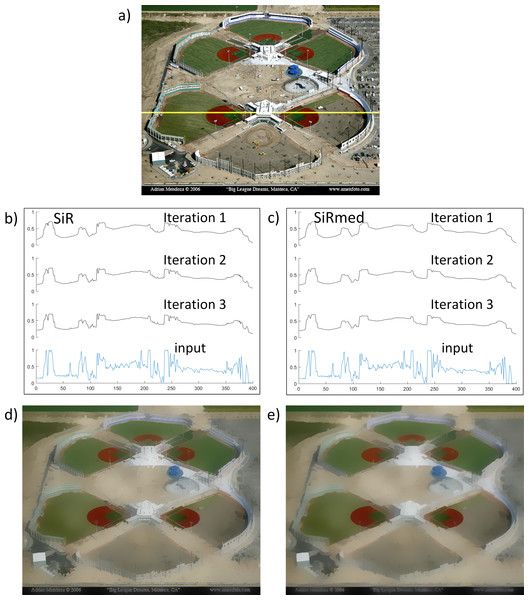Figure 1: Effects of SiR and SiRmed filtering on image intensity. (A) Input image (Photo credit: Adrian Mendoza). (B, C) 1D Image intensity distribution along a horizontal cross section of (A) (yellow line) after the first 3 iterations of respectively SiR and SiRmed. The lowest curves show the original intensity distribution in (A). (D, E) Results after 5 iterations of respectively SiR and SiRmed. The spatial and range kernels used in the bilateral filters for this example were respectively σ spatial = 5 and σ range = 0 . 05 .   Download full-size image DOI: 10.7717/peerjcs.72/fig-1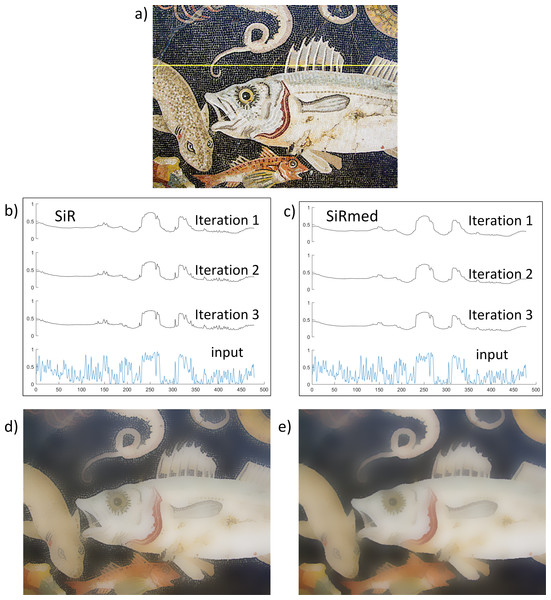Figure 2: Effects of SiR and SiRmed filtering on image intensity. (A) Input image. (B, C) 1D Image intensity distribution along a horizontal cross section of (A) (yellow line) after the first 3 iterations of respectively SiR and SiRmed. The lowest curves show the original intensity distribution in (A). (D, E) Results after 5 iterations of respectively SiR and SiRmed. The spatial and range kernels used in the bilateral filters for this example were respectively σ spatial = 5 and σ range = 0 . 05 .   Download full-size image DOI: 10.7717/peerjcs.72/fig-2

### Relative performance of SiR and SiRmed

Figures 1 and 2 illustrate the effect of including a median filter with a kernel size of 3 × 3 at the end of each iteration step in the SiR framework (see Table 2). Figure 1 shows the original input image (a) with the result of respectively SiR (Fig. 1D) and SiRmed (Fig. 1E) after 5 iterations. In addition, this figure also shows the 1D image intensity distribution along a horizontal cross section of the input image (yellow line in Fig. 1A) after each of the first 3 iterations of both SiR (Fig. 1C) and SiRmed (Fig. 1D). The lowest curves represent the original intensity distribution (Fig. 1A). Comparison of Figs. 1D and 1E shows that SiRmed effectively removes small details all over the image, while SiR reintroduces small details near large scale edges (notice for instance the light poles all over the stadium, that are restored in Fig. 1D but are nicely removed in Fig. 1E). A comparison of Figs. 1B and 1C shows that SiR indeed restores high frequency details near large edge discontinuities, while SiRmed restores these large scale edges without the reintroduction of small scale details. This effect is also clearly demonstrated in Fig. 2, where SiR fails to remove the small scale details all around the outlines of the tentacles and the fishes, while SiRmed effectively filters small elements all around these larger objects while both preserving their outlines and smoothing their interior.

### Relative performance of RGF, SiRmed and AGF

Figure 3 shows the results of RGF, SiRmed and AGF filtering after the first 3 iteration steps. Notice that all three filter schemes iteratively restore large scale image edges proceeding from an initial low resolution version of the input image. In this process RGF gradually smoothes the curvature of the large scale edges, while SiRmed smoothes the overall image intensity resulting in a global contrast reduction. In contrast, AGF restores the large scale edges without smoothing their curvature and with preservation of local image contrast. Figures 4B4D illustrate these effects by showing 1D horizontal cross sections. The contrast reducing effect of SiR is clearly shown in Fig. 4C as an overall reduction of the height of the large scale peaks. Comparison of Figs. 4E and 4G illustrates that RGF smoothes the curvature of large scale edges while AGF preserves edge curvature.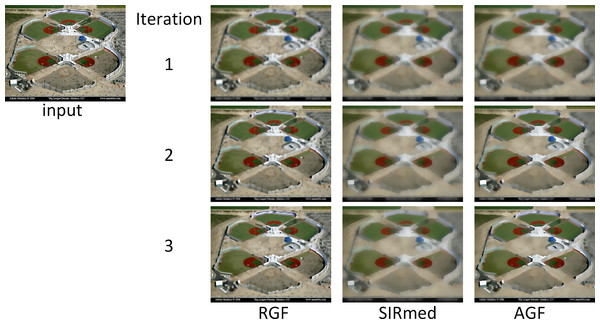Figure 3: Results of RGF, SiRmed and AGF filtering for the first 3 iteration steps. The spatial and range kernels used in the bilateral filters for this example were respectively σ spatial = 5 and σ range = 0 . 05 . left. (Photo credit: Adrian Mendoza).   Download full-size image DOI: 10.7717/peerjcs.72/fig-3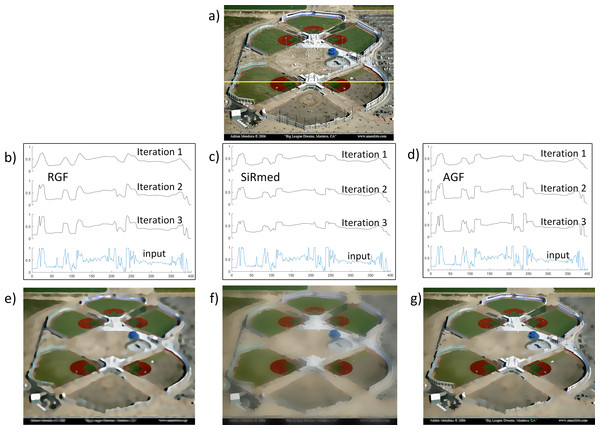Figure 4: Comparison of RGF, SiRmed and AGF filtering. (A) Input image (Photo credit: Adrian Mendoza). (B–D) 1D Image intensity distribution along a horizontal cross section (yellow line in (A)) after 3 successive iterations. (E–G) Results after 5 iteration steps. The spatial and range kernels used in the bilateral filters for this example were respectively σ spatial = 5 and σrange = 0.05.   Download full-size image DOI: 10.7717/peerjcs.72/fig-4

Figure 5 shows the details that are removed by filtering with respectively RGF (Fig. 5B), SiRmed (Fig. 5C) and AGF (Fig. 5D) after 5 iterations, together with the original input image (Fig. 5A). These images are obtained by subtracting the original image from the filtered ones and computing the mean of the absolute value across the three color channels. This example illustrates that each of the three filters removes small scale details while preserving the larger scale edges to a certain extent. Note that AGF removes small image details all over the image support, while the original large scale edges (e.g., the outlines of the temple) remain relatively unaffected. In contrast, both RGF and SiRmed significantly alter the articulation of the original large scale edges. RGF smoothes the local edge curvature while SiRmed affects the local mean image intensity (resulting in local contrast reduction). As a result, large scale image contours (e.g., the outlines of the temple) are clearly visible in Figs. 5B and 5C, and much less pronounced in Fig. 5D.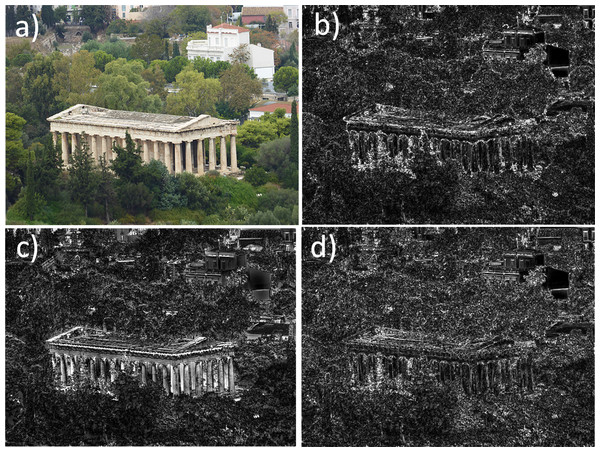Figure 5: The elimination of small scale details by RGF, SiRmed and AGF filtering. Absolute difference between input image (A) and the results of respectively RGF (B), SiRmed (C) and AGF (D) after 5 iterations. The images have been enhanced for visual display. The spatial and range kernels used in the bilateral filters for this example were respectively σ spatial = 5 and σ range = 0 . 05 .   Download full-size image DOI: 10.7717/peerjcs.72/fig-5

Figure 6 further illustrates the differential performance of all four filters investigated here (AGF, RGF, SiR, and SiRmed) on an artificial image with noise. Figure 6A shows some simple geometric shapes (cross, triangle, square and wheel) with different sizes on a step-edge background with 30% additional Gaussian noise. Figures 6B6E show the results of respectively AGF, RGF, SiR and SiRmed filtering of Fig. 6A after five iteration steps. This example shows that SiR restores noise near the edges of larger details and along the vertical step edge in the background, while AGF, RGF and SiRmed effectively reduce noise all over the image plane. Also, RGF (Fig. 6C) smoothes edge curvature, in contrast to AGF, SiR and SiRmed which retain the original edge curvature. This can for instance be seen in Fig. 6C where the sharp edges of the crosses, the corners of the triangles and squares, and the spokes of the wheels are all rounded after filtering. This example also illustrates that both SiR (Fig. 6D) and SiRmed (Fig. 6E) effectively reduce image contrast due to the intensity smoothing that is inherent in this method. AGF does not introduce any halos. In contrast, RGF produces high intensity halos, and SiR and SiRmed both produce halos with a large spatial extent (see Fig. 6C near the crosses and wheels).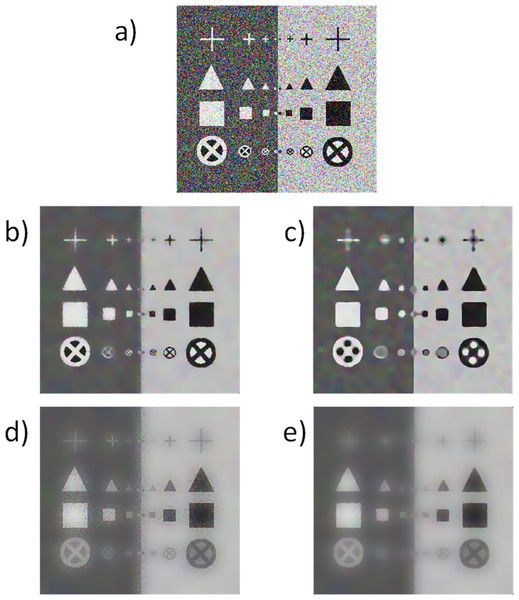Figure 6: Comparison of AGF, RGF, SiR and SiRmed filtering. (A) Artificial input image with 30% Gaussian noise added. Results of (B) AGF, (C) RGF, (D) SiR and (E) SiRmed filtering after 5 iteration steps. The spatial and range kernels used in the bilateral filters for this example were respectively σ spatial = 5 and σ range = 0 . 05 .   Download full-size image DOI: 10.7717/peerjcs.72/fig-6

### Effects of different parameter settings

The results of RGF, SiRmed and AGF for different values of the parameters σs and σr are shown in Figs. 79. In these figures rows correspond to different amounts of domain filtering (i.e., different values of σs) and columns to different amounts of range filtering (i.e., different values of σr). These figures show that large spatial kernels and large range kernels both result in blurred image representations. Large spatial kernels cause averaging over large image areas, while large range kernels cause averaging over a wider range of image values.

In RGF (Fig. 7) the smoothing of large scale edge curvature increases with increasing σs. This effect can for instance clearly be seen in the first column of Fig. 7 (for σr = 0.05) where the curves in the roof of the temple just above the pillars are still visible for σs = 3, reduced for σs = 6 and removed for σs = 9.

In SiRmed (Fig. 8) the reduction of local image contrast increases with increasing σs. This effect can for instance clearly be seen in the first column of Fig. 8 (for σr = 0.05) where the contrast in the background vegetation around the temple is still visible for σs = 3, reduced for σs = 6 and almost removed for σs = 9.

Figure 9 shows the effects of different parameter settings on the performance of the proposed AGF framework. In contrast to RGF, AGF preserves large scale edge curvature, as can be seen in the first column of Fig. 9 (for σr = 0.05). Notice that the curves in the roof of the temple just above the pillars remain unaffected, even for the largest size of the spatial kernel (σs = 9). In contrast to SiRmed, AGF also preserves local image intensity (contrast).

### Runtime evaluation

In this study we used a Matlab implementation of the RGF written by Zhang et al. (2014) that is freely available from the authors (at http://www.cs.cuhk.edu.hk/ leojia/projects/rollguidance). The JBF, which is part of this code, is also used here to implement both the SiR filter (see Bilateral filter') and the AGF filter (see Alternating Guided Filter'). We made no effort to optimize the code of the algorithms. We conducted a runtime test on a Dell Latitude laptop with an Intel i5 2 GHz CPU and 8 GB memory. The algorithms were implemented in Matlab 2016a. Only a single thread was used without involving any SIMD instructions. For this test we used a set of 22 natural RGB images (also provided in the Supplemental Information with this paper). The spatial and range kernels used in the bilateral filters for this test were fixed at respectively ${\sigma }_{\mathrm{spatial}}=5$ and ${\sigma }_{\mathrm{range}}=0.05$, and the number of iterations was set to 5. The mean runtimes for AGF, RGF, SiR and SiRmed were respectively 3.80 ± 0.27, 1.64 ± 0.12, 1.80 ± 0.14 and 2.17 ± 0.16 s. This shows that the mean runtime of AGF is about equal to the sum of the runtimes of RGF and SiRmed. This result is as expected, since the steps involved in AGF are a combination of the steps involved in both RGF and SiRmed.

## Conclusions

In this paper we presented the new edge-preserving Alternating Guided Filter (AGF) smoothing filter that eliminates small scale image details while preserving both the articulation and curvature of large scale edges and local mean image intensity. The AGF framework integrates the recently introduced RGF and (a slightly adapted version of) SiR filters in an alternating iterative scheme. AGF combines the large scale edge and local intensity preserving image smoothing properties of the RGF with the large scale edge restoring properties of the SiR. However, it does not suffer from the drawbacks of both of its components: the curvature smoothing of large scale edges by RGF and the local intensity reduction and restoration of small scale details near large scale edges by SiR. The AGF is simple to implement and efficient. Application to a wide range of images has shown that AGF consistently produces high-quality results. Possible applications areas for AGF are for instance detail enhancement, denoising, edge extraction, JPEG artifact removal, multi-scale structure decomposition, saliency detection etc. (see e.g., He, Sun & Tang, 2013; Kniefacz & Kropatsch, 2015; Zhang et al., 2014).

## Supplemental Information

### Competing Interests

The author declares there are no competing interests.

### Author Contributions

Alexander Toet conceived and designed the experiments, performed the experiments, analyzed the data, contributed reagents/materials/analysis tools, wrote the paper, prepared figures and/or tables, performed the computation work, reviewed drafts of the paper.

### Data Availability

The following information was supplied regarding data availability:

The raw data has been supplied as Supplemental Dataset.

### Funding

This work was supported by the Air Force Office of Scientific Research, Air Force Material Command, USAF, under grant number FA9550-15-1-0433. The funders had no role in study design, data collection and analysis, decision to publish, or preparation of the manuscript.